The two types of value-based methods

But what means acting according to our policy? We don’t have a policy in value-based methods since we train a value-function and not a policy?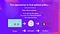The policy takes a state as input and outputs what action to take at that state (deterministic policy).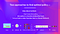Given a state, our action-value function (that we train) outputs the value of each action at that state, then our greedy policy (that we defined) selects the action with biggest state-action pair value.

The State-Value function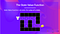If we take the state with value -7: it’s the sum of Expected return starting at that state and taking actions according to our policy (greedy policy) so right, right, right, down, down, right, right.

The Action-Value function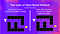Note: We didn’t fill all the state-actions pair for the example of Action-value function

The Bellman Equation: simplify our value estimation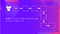To calculate the value of State 1: the sum of rewards if the agent started in that state, and then followed the greedy policy (taking actions that leads to the best states values) for all the time steps.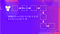To calculate the value of State 2: the sum of rewards if the agent started in that state, and then followed the policy for all the time steps.To calculate the value of State 1: the sum of rewards if the agent started in that state 1, and then followed the policy for all the time steps.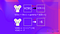For simplification here we don’t discount so gamma = 1.

Summary

Building next-gen AI in games using NLP and Deep RL 🧠🕹️ | Founder Deep Reinforcement Learning course 📚 bit.ly/34fMhwc |

More from Thomas Simonini

Building next-gen AI in games using NLP and Deep RL 🧠🕹️ | Founder Deep Reinforcement Learning course 📚 bit.ly/34fMhwc |